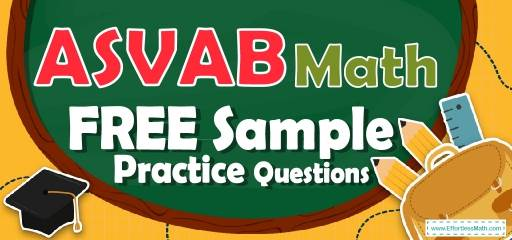# ASVAB Math FREE Sample Practice QuestionsPreparing for the ASVAB Math test? To do your best on the ASVAB Math test, you need to review and practice real ASVAB Math questions.  There’s nothing like working on ASVAB Math sample questions to hone your math skills and put you more at ease when taking the ASVAB Math test. The sample math questions you’ll find here are brief samples designed to give you the insights you need to be as prepared as possible for your ASVAB Math test.

Check out our sample ASVAB Math practice questions to find out what areas you need to practice more before taking the ASVAB Math test!

Start preparing for the 2022 ASVAB Math test with our free sample practice questions. Also, make sure to follow some of the related links at the bottom of this post to get a better idea of what kind of mathematics questions you need to practice.

## 10 Sample ASVAB Math Practice Questions

1- In the following diagram what is the value of $$x$$?
$$\img{https://appmanager.effortlessmath.com/public/images/questions/q2.JPG}$$

A. $$60^\circ$$

B. $$90^\circ$$

C. $$45^\circ$$

D. $$15^\circ$$

2- In the following right triangle, what is the value of $$x$$ rounded to the nearest hundredth?
$$\img{https://appmanager.effortlessmath.com/public/images/questions/q3.JPG}$$

A. 23.24

B. 2.33

C. 10.29

D. 6.40

3- $$(5x + 5) (2x + 6) =$$ ?

A. $$5x + 6$$

B. $$10x^2 + 40x + 30$$

C. $$5x + 5x + 30$$

D. $$5x^2 + 5$$

4- $$5 (a – 6) = 22$$, what is the value of a?

A. 2.4

B. 10.4

C. 7

D. 11

5- If $$3^{24}=3^8× 3^x$$, what is the value of $$x$$?

A. 2

B. 1.5

C. 3

D. 16

6- Which of the following is an obtuse angle?

A. $$116^\circ$$

B. $$80^\circ$$

C. $$68^\circ$$

D. $$25^\circ$$

7- Factor this expression: $$x^2 + 5 − 6$$

A. $$x^2(5 + 6)$$

B. $$x(x + 5 – 6)$$

C. $$(x + 6)(x – 1)$$

D. $$(x + 6)(x – 6)$$

8- Find the slope of the line running through the points (6, 7) and (5, 3).

A. $$\frac{1}{4}$$

B. 4

C. $$-4$$

D. $$-\frac{1}{4}$$

9- What is the value of $$\sqrt {100} × \sqrt{36}$$?

A. 120

B. $$\sqrt{136}$$

C. 60

D. $$\sqrt{16}$$

10- Which of the following is not equal to 52?

A. the square of 5

B. 5 squared

C. 5 cubed

D. 5 to the second power

## Best ASVAB Math Prep Resource for 2022

1- C
All angles in a triable add up to 180 degrees.
$$90^\circ + 45^\circ = 135^\circ$$
$$x = 180^\circ − 135^\circ = 45^\circ$$

2- D
Use Pythagorean Theorem: $$a^2 + b^2 = c^2$$
$$(4)^2 + (5)^2 = c^2 ⇒ 16 + 25 = 41 = C^2$$
$$C = \sqrt{41} = 6.403$$

3- B
Use FOIL (first, out, in, last) method.
$$(5x + 5) (2x + 6) = 10x^2 + 30x + 10x + 30 = 10x^2 + 40x + 30$$

4- B
$$5(a–6)=22 ⇒ 5a-30=22 ⇒5a=22+30=52$$
$$⇒ 5a=52⇒a=\frac{52}{5}= 10.4$$

5- D
Use exponent multiplication rule:
$$x^a . x^b = x^{a + b}$$
Then:
$$3^{24}=3^8× 3^x=3^{8+x}$$
$$24 = 8 + x ⇒x=24-8=16$$

6- A
An obtuse angle is an angle of greater than 90 degrees and less than 180 degrees. The only choice A is an obtuse angle.

7- C
To factor the expression $$x^2 + 5 – 6$$, we need to find two numbers whose sum is 5 and their product is $$-6$$.
Those numbers are 6 and $$-1$$. Then:
$$x^2+5-6=(x+6)(x-1)$$

8- B
Slope of a line:$$\frac{y_2- y_1}{x_2 – x_1}=\frac{rise}{run}$$
$$\frac{y_2- y_1}{x_2 – x_1}=\frac{3- 7}{5 – 6}=\frac{-4}{-1}= 4$$

9- C
$$\sqrt{100} = 10, \sqrt{36} = 6$$
$$10 × 6 = 60$$

10- C
Only choice c is not equal to $$5^2$$

Looking for the best resource to help you succeed on the ASVAB Math test?

## More from Effortless Math for ASVAB Test …

### Looking for a FREE online ASVAB Math course to help you learn all ASVAB Mathematics topics?

Check out The Ultimate ASVAB Math Course.

Use our 5 Best ASVAB Math Study Guides to help you prepare well for the ASVAB Math Test.

## Have any questions about the ASVAB Test?

### What people say about "ASVAB Math FREE Sample Practice Questions - Effortless Math: We Help Students Learn to LOVE Mathematics"?

No one replied yet.

X
52% OFF

Limited time only!

Save Over 52%

SAVE $40 It was$76.99 now it is \$36.99